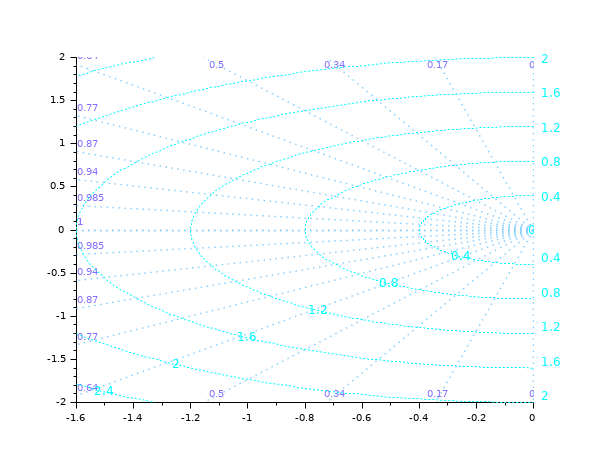Scilab Home page | Wiki | Bug tracker | Forge | Mailing list archives | ATOMS | File exchange
Change language to: English - Français - Português - 日本語 -
Справка Scilab >> CACSD > Linear Analysis > Stability > sgrid

# sgrid

draws a s-plane grid

### Syntax

```sgrid()
sgrid(zeta, wn)
sgrid(.., colors)
sgrid(.., "new")```

### Arguments

zeta

vector of damping factors. Only values in `[0 1]` are taken into account. The default values are ~ cosd(90:-10:0) = `[0 0.17 0.34 0.5 0.64 0.77 0.87 0.94 0.985 1]`.

wn

array of natural frequencies in Hz. only positive values are taken into account. If not given it is computed by the program to fit with the boundaries of the plot.

colors

a scalar or a vector with 2 elements [circles_col, rays_col], specifying the color(s) of circles and rays of the frame, and their labels: predefined colors names (like "red"), or colors hexadecimal codes (like "#34DDFA"), or colors indices in the current colormap are accepted.

"new"

This option clears all contents of the current axes before plotting the grid. It may be specified at any position among input arguments.

### Description

The `sgrid` function is often used to draw a grid for Evans root locus of continuous time linear systems. In such a case the `sgrid` function should be called after the call to evans. For discrete time linear systems one should use zgrid function instead.

`sgrid` plots curves of constant damping ratio at values given by `zeta`, and constant natural frequency at values given by `wn`.

The `colors` argument may be used to assign a color for constant damping ratio curves (`colors(2)`) and for constant natural frequency curves (`colors(1)`).

`sgrid(), sgrid("new"), sgrid(colors)` or `sgrid(colors, "new")` plots a default grid.

### Examples

```clf
subplot(1,2,1), sgrid([0:0.1:0.9 0.97 1], 0.5:0.5:3)
subplot(1,2,2), sgrid("grey70")```Post-tuning graphical elements of the grid:

```sgrid()

sGrid = gca().children.children.children;
i = find(sGrid(3:\$).type=="Polyline" & sGrid(1:\$-2).type=="Polyline",1);

Circles = sGrid(1:i-1);
Circ_text = Circles(Circles.type=="Text");      // Labels
Circ_text.font_size = 2;
Circ_lines = Circles(Circles.type=="Polyline"); // Circles
Circ_lines.line_style = 8;

Rays = sGrid(i:\$);
Rays(Rays.type=="Text").font_foreground = color("light slate blue");
Rays_lines = Rays(Rays.type=="Polyline");
set(Rays_lines, "line_style", 9, "thickness", 1.5);```Evans plot + a s grid:

```H = syslin('c',352*poly(-5,'s')/poly([0,0,2000,200,25,1],'s','c'));
clf
evans(H,100)
sgrid()```### History

 Версия Описание 6.0.2 colors can be specified by their names or by their #RRGGBB code